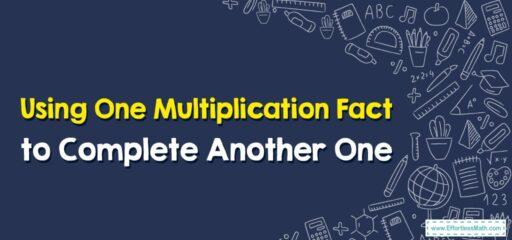# How to Use One Multiplication Fact to Complete Another One

Knowing one multiplication fact can help you quickly figure out related facts. This strategy is often used in elementary mathematics education and is based on the principles of commutative, associative, and distributive properties of multiplication.## A Step-by-step Guide to Using One Multiplication Fact to Complete Another One

Sure, using one multiplication fact to complete another is a useful strategy when learning multiplication. It is based on the properties of multiplication like the Commutative, Associative, and Distributive properties. Here is a step-by-step guide:

### Step 1: Identify Known Multiplication Facts

First, you’ll want to start with a multiplication fact that you already know. For example, let’s say you know that $$5 x 4 = 20$$.

### Step 2: Using the Commutative Property

The Commutative Property states that you can switch the order of the factors and the product remains the same. So if you know that $$5 x 4 = 20$$, you also know that $$4 x 5 = 20$$.

### Step 3: Using the Associative Property

The Associative Property allows us to change the grouping of numbers in a multiplication problem without changing the product. For example, if you know that $$2 x 4 = 8$$, you can use this to find out what $$4 x 4$$ is. Because 4 is the same as $$2 x 2$$, you can group the problem like this: $$2 x (2 x 4)$$. Then, using the fact you already know $$(2 x 4 = 8)$$, you can simplify it to $$8 x 2 = 16$$.

### Step 4: Using the Distributive Property

The Distributive Property allows you to break down a larger multiplication problem into smaller, more manageable parts. For example, if you want to figure out what $$6 x 7$$ is, but you only know up to your 5 times table, you can break 6 down into $$5 + 1$$. Then, use the Distributive Property to rewrite the problem as $$(5 x 7) + (1 x 7)$$. If you know that $$5 x 7 = 35$$ and $$1 x 7 = 7$$, you can add these together to get 42.

So, by using these properties of multiplication, you can leverage multiplication facts you already know to figure out new multiplication facts. This is a great way to build your understanding and fluency with multiplication.

### What people say about "How to Use One Multiplication Fact to Complete Another One - Effortless Math: We Help Students Learn to LOVE Mathematics"?

No one replied yet.

X
30% OFF

Limited time only!

Save Over 30%

SAVE $5 It was$16.99 now it is \$11.99